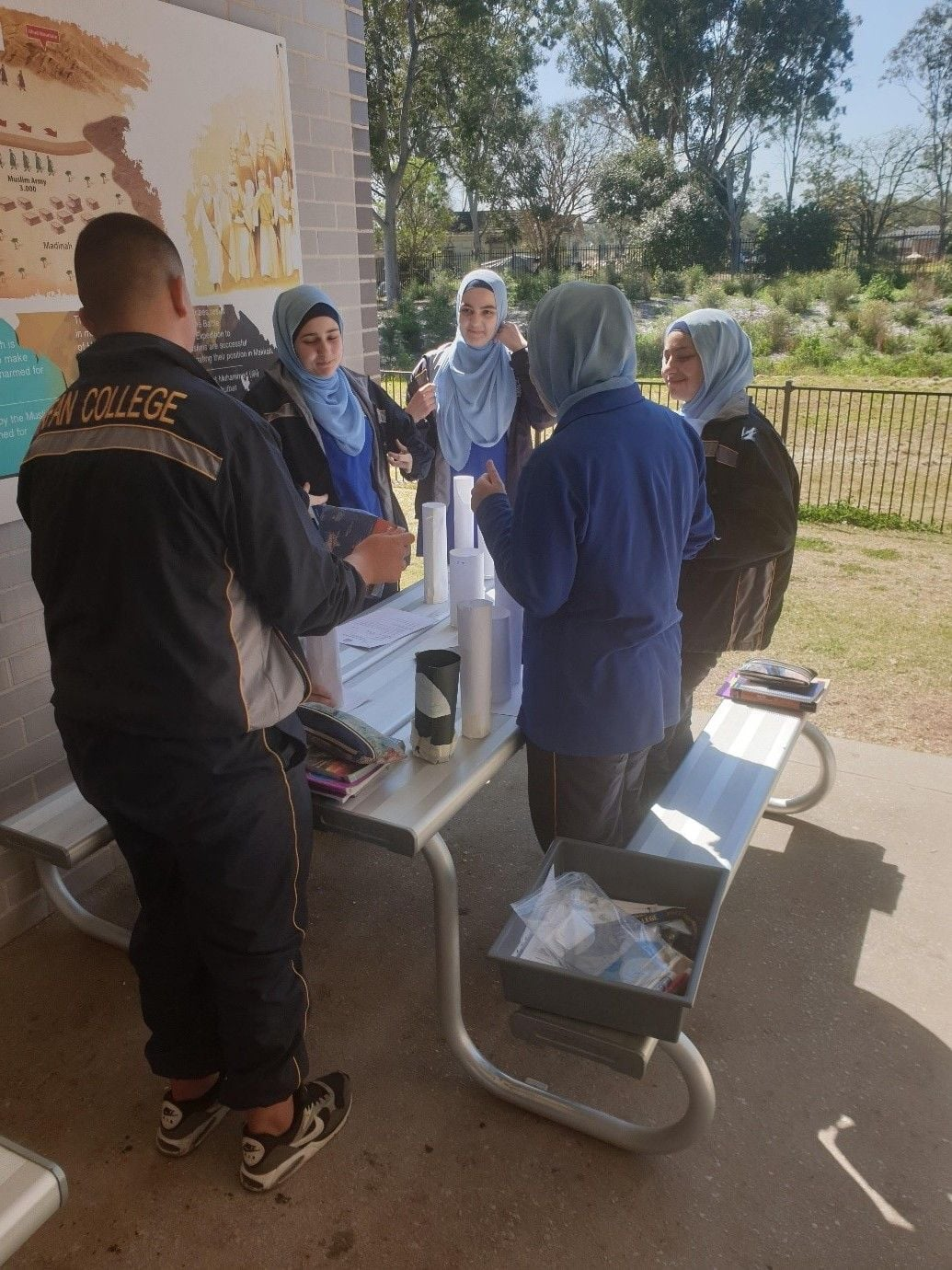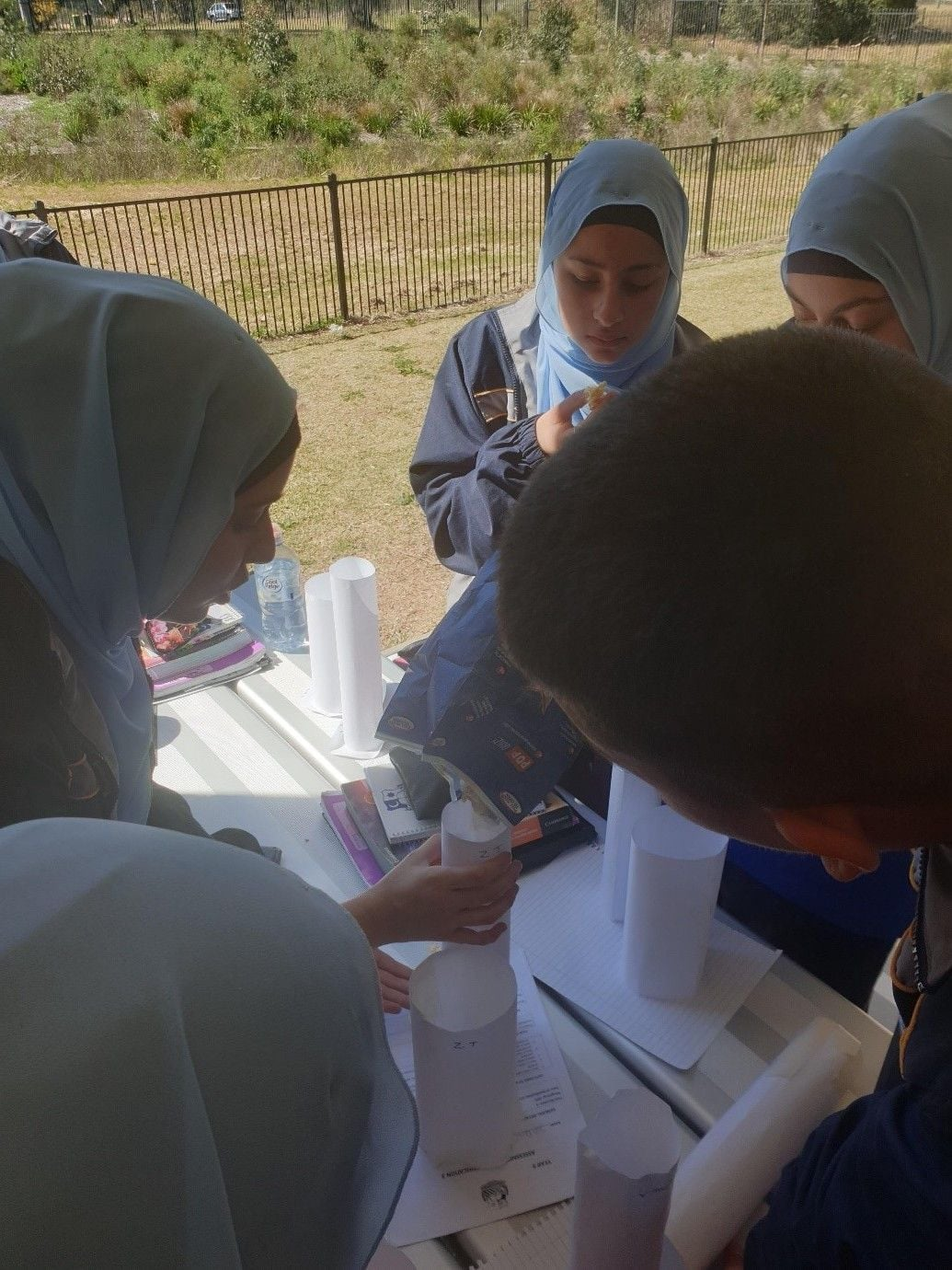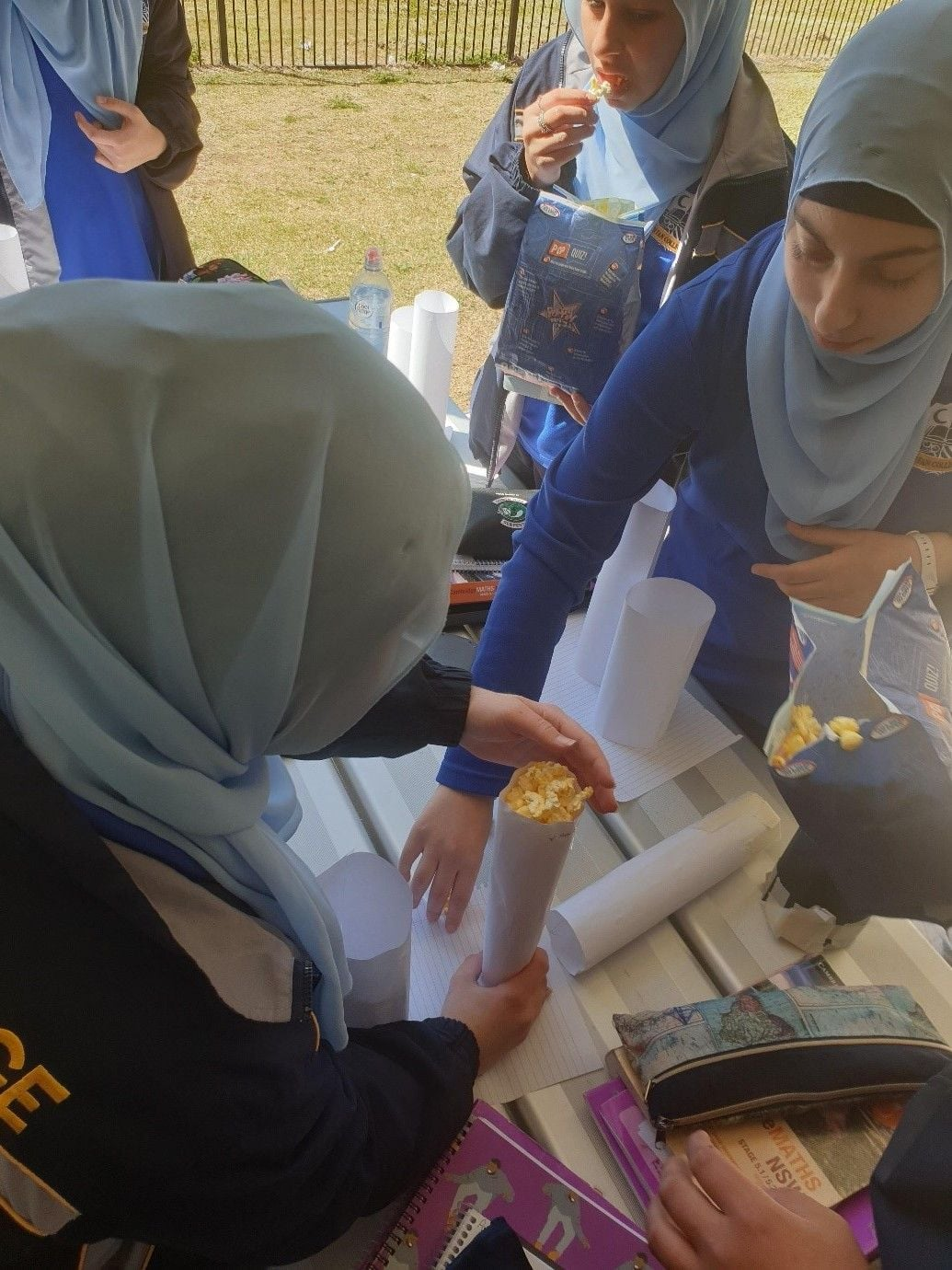Home >  Blog >  Mathematic T3 2019

# Mathematic T3 2019

Posted by Joumana Issa on 14 October 2019
Assalama Alaykum Wa Rahmatullah Wa Barakatu,
This term our secondary mathematics students completed assignment for their assessment tasks.
• The Year 7 students studied the following topics: Computation with decimals and fractions, Time (including time zones), Algebraic techniques and Equations. They completed a Probability Assignment where they needed to differentiate between Theoretical and Experimental probability.
• The Year 8 students studied the following topics:  Ratio and Rates, Angle relationships and properties of geometrical figures and Linear relationships. They completed an assignment based on the Golden Ratio and its existence in nature
• The Year 9/5.2 students studied the following topics: Algebraic Expressions and Equations, Right-Angled Triangles, Linear Relationships, Rates and Ratios. They also worked on Measurement such as Surface area, Volume and Capacity.
• The Year 9/5.3 students studied the following topics: Length, Area, Surface Area and Volume, Indices & Surds, Properties of Geometrical Figures. They also worked on an assignment based on Volume and capacity.
• The Year 10/5.2 students studied the following topics: Single Variable Data Analysis and Bi-Variate Data Analysis, Linear relationships, Properties of geometrical figures and Right-angled triangles. They completed an assignment based on their survey where they needed to collate the data to determine five number summaries and graphs.
• The Year 10/5.3 students studied the following topics: Algebraic Techniques, Equations and Linear Relationships, Geometrical Figures and Circle Geometry and Trigonometry. They also completed an assignment based on higher order Algebra and Equations.
Overall our students did very well and many of them achieved 100%. Most importantly they valued the experience gained from completing these assignments which subsequently helped them enhance the learning outcomes.

The pictures below show the Year 9/5.3 Mathematics students trying to determine which solid held more popcorn, however they needed to determine the result prior to the experiment. They were also comparing the volume of two cylinders created using the SAME two sheets of paper.Author:Joumana Issa
 Tags:Mathematics - Secondary

## Stay up to date

with news & important announcements!

### Sitemap

2089 – 2109 Elizabeth Drive,
Cecil Park NSW 2178

### Opening Hours

Mon – Fri: 8:00am – 4:00pm

(02) 9826 2885

### Email

office@irfancollege.nsw.edu.au